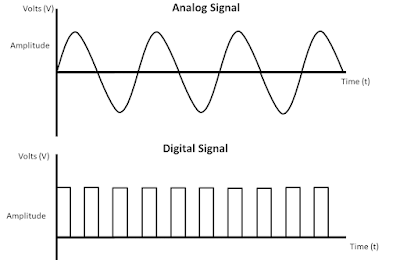## Posts

Showing posts with the label signal

### What types of devices provide analog signals?

What types of devices provide analog signals?  Basically devices like control valve, PT, LT, TT, %LEL, O2, load cell provide analog signal.

### Analog Signal VS Digital SignalAnalog Signal VS Digital Signal Signal A signal is basically the transmission of information from one side to another and this information is electrical form. And signal is a way of communication and represents information or data. A signal is the carrying of information through an electromagnetic wave. Basically signal is the conversion of data into electromagnetic signal and this signal is analog or digital. And based on this format the signals are divided into two categories. And this type is analog signal and digital signal. A signal is an electrical current and is used to carry data from one system to another. Analog Signal An analog signal is a continuous signal and an analog signal represents the equivalent of another quantity. Basically an analog signal is a continuous electrical signal. Analog signals are used to carry information. Basically this signal is represented by a sine wave and this signal has a continuous range of values. Generally analog signals are used only in ana

### Analog signal

Analog Signal An analog signal is a continuous signal and an analog signal represents the equivalent of another quantity. Basically an analog signal is a continuous electrical signal. Analog signals are used to carry information. Basically this signal is represented by a sine wave and this signal has a continuous range of values. Generally analog signals are used only in analog devices. Examples of analog signals are natural sounds, human voices, analog phones, old land line telephones, etc. Basically instrumentation devices use 4 to 20 mA or 0 to 20 mA signals. And this instrument or controller keeps this voltage constant and takes it as current signal. An analog signal is a form of electrical energy such as voltage, current. Analog signal is more accurate than digital signal and analog signal has infinite memory. Analog signals produce more noise and analog signals have lower bandwidth